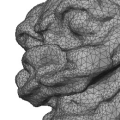CGAL 4.14 - Poisson Surface Reconstruction
Poisson Surface Reconstruction ReferencePierre Alliez, Laurent Saboret, Gaël Guennebaud
This package implements a surface reconstruction method: Poisson Surface Reconstruction. It takes as input a set of points with oriented normals and computes an implicit function. The CGAL surface mesh generator can then be used to extract an iso-surface from this function.
Introduced in: CGAL 3.5
Depends on: CGAL and Solvers
BibTeX: cgal:asg-srps-19a
Windows Demo: Polyhedron demo
Common Demo Dlls: dlls

## Classes

• CGAL::Poisson_reconstruction_function<GeomTraits>

## Classes

class  CGAL::Poisson_reconstruction_function< Gt >
Implementation of the Poisson Surface Reconstruction method. More...

## Functions

template<typename PointInputIterator , typename PointMap , typename NormalMap , typename PolygonMesh , typename Tag = CGAL::Manifold_with_boundary_tag>
bool CGAL::poisson_surface_reconstruction_delaunay (PointInputIterator begin, PointInputIterator end, PointMap point_map, NormalMap normal_map, PolygonMesh &output_mesh, double spacing, double sm_angle=20.0, double sm_radius=30.0, double sm_distance=0.375, Tag tag=Tag())
Performs surface reconstruction as follows: More...

## ◆ poisson_surface_reconstruction_delaunay()

template<typename PointInputIterator , typename PointMap , typename NormalMap , typename PolygonMesh , typename Tag = CGAL::Manifold_with_boundary_tag>
 bool CGAL::poisson_surface_reconstruction_delaunay ( PointInputIterator begin, PointInputIterator end, PointMap point_map, NormalMap normal_map, PolygonMesh & output_mesh, double spacing, double sm_angle = 20.0, double sm_radius = 30.0, double sm_distance = 0.375, Tag tag = Tag() )

#include <CGAL/poisson_surface_reconstruction.h>

Performs surface reconstruction as follows:

• compute the Poisson implicit function, through a conjugate gradient solver, represented as a piecewise linear function stored on a 3D Delaunay mesh generated via Delaunay refinement
• meshes the function with a user-defined precision using another round of Delaunay refinement: it contours the isosurface corresponding to the isovalue of the median of the function values at the input points
• outputs the result in a polygon mesh

This function relies mainly on the size parameter spacing. A reasonable solution is to use the average spacing of the input point set (using compute_average_spacing() for example). Higher values increase the precision of the output mesh at the cost of higher computation time.

Parameters sm_angle, sm_radius and sm_distance work similarly to the parameters of SurfaceMeshFacetsCriteria_3. The latest two are defined with respect to spacing.

Template Parameters
 PointInputIterator is a model of InputIterator. PointMap is a model of ReadablePropertyMap with value type Point_3. NormalMap is a model of ReadablePropertyMap with value type Vector_3. PolygonMesh a model of MutableFaceGraph with an internal point property map. Tag is a tag whose type affects the behavior of the meshing algorithm (see make_surface_mesh()).
Parameters
 begin iterator on the first point of the sequence. end past the end iterator of the point sequence. point_map property map: value_type of InputIterator -> Point_3. normal_map property map: value_type of InputIterator -> Vector_3. output_mesh where the reconstruction is stored. spacing size parameter. sm_angle bound for the minimum facet angle in degrees. sm_radius bound for the radius of the surface Delaunay balls (relatively to the average_spacing). sm_distance bound for the center-center distances (relatively to the average_spacing). tag surface mesher tag.
Returns
true if reconstruction succeeded, false otherwise.
Examples:
Poisson_surface_reconstruction_3/poisson_reconstruction_function.cpp.